DDG（离散微分几何）：曲面参数化 | HyperPlane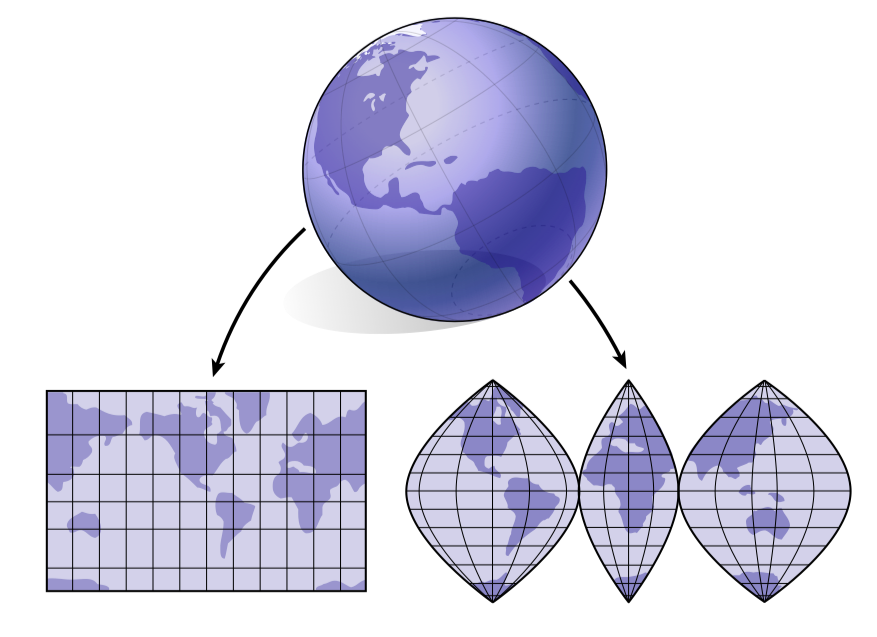0.1 两个四分之一的旋转得到翻转：复数的一个简要介绍 如果你看到过复数，你可能遇到过像-1的“平方根”这样对于虚数单位$i$完全糟糕的描述：

$i = \sqrt{-1}$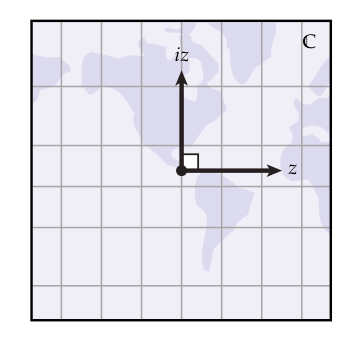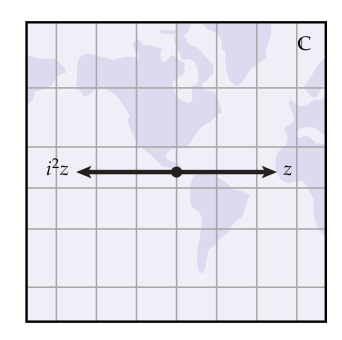$i^2 = -1$

## 共形结构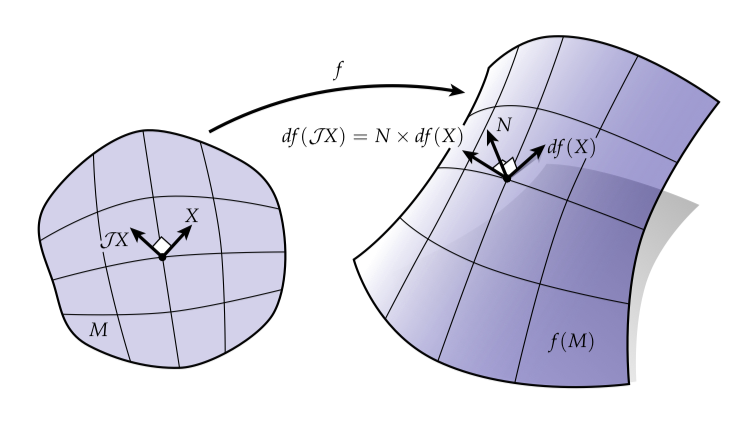$N \times df(X)$

$df(\mathcal{J}X) = N \times df(X)$

$\mathcal{J}^2 = -id$

## 柯西-黎曼方程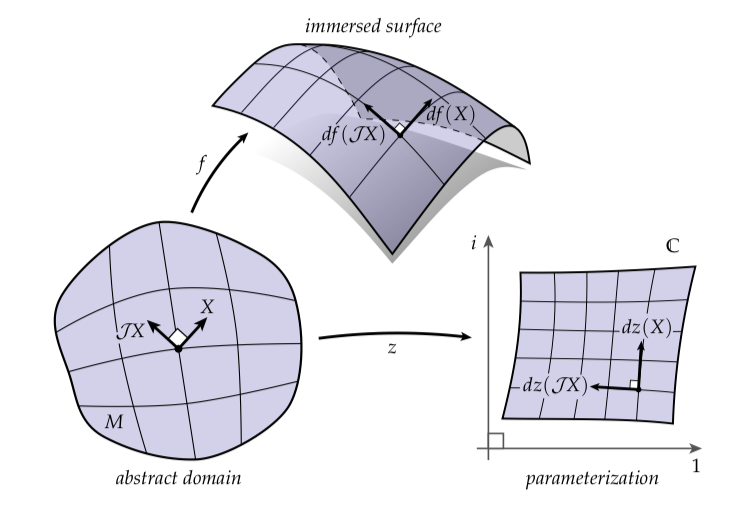$dz(\mathcal{J}X) = idz(X)$

## 黎曼曲面上的微分形式

• 0-型看起来像标量函数
• 1-型看起来像向量场
• 2-型看起来像标量乘面积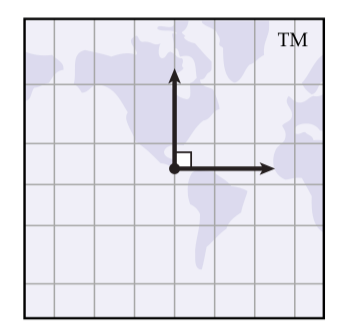$\star \alpha (X) \coloneqq \alpha (\mathcal{J}X)$

$\star \omega \coloneqq \omega(X, \mathcal{J}X)$

$\| \alpha \| =\coloneqq \sqrt{\langle\langle \alpha, \alpha \rangle\rangle }$

$(\bar{\alpha})(X) \coloneqq \overline{(\alpha(X))}$

## 共形参数化

$dz(\mathcal{J}X) = idz(X)$

$\star dz = idz$

$E_C(z) \coloneqq \frac{1}{4} \| \star dz - idz \|^2$

$\min_z E_C(z)$

$\min_z \| \star dz - idz \|^2$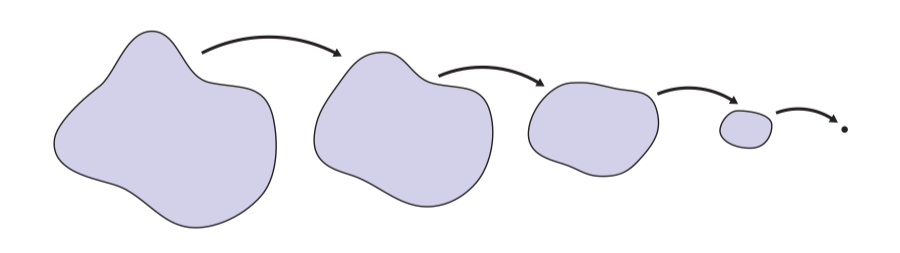$\min_z E_C(z) = \frac{1}{2} \langle\langle \Delta z, z\rangle\rangle - \mathcal{A}(z)$

$\Delta z = 0$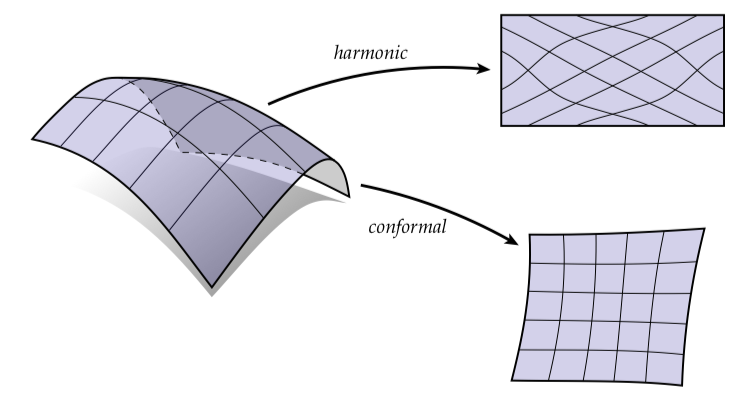\begin{aligned} \min_z & E_C(z) \\ s.t. & \| z \| = 1, \\ & \langle\langle z, \mathbb{1} \rangle\rangle = 0 \end{aligned}

$\frac{1}{A} \intop_M zdA = 0$